# Discussion

In the chosen example several different approaches were taken to a single, simple problem. The key issue being considered here is that the form in which the answer is available depends on the approach taken to solving the problem. There is a mathematical function, some pseudo sample data, an asymptotic expansion, and a numerical approximation. The rest of this thesis is concerned with presentation methods which may be used across all of these implementations.

Note that each of the above approaches gives a similar answer to the problem. The following summarises the posterior densities obtained for the posterior mean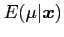and posterior varianceby each method:

Table:Results for posterior densities for manufacturing problem.
 Mean Variance Analytic 3.508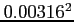Gibbs Sampler 3.507999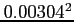Predictive mean and variance 3.508014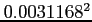Asymptotic 3.508Bayes4 Normal Prior 3.50800Bayes4 t Prior 3.50800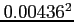Note that if the posterior mean is used as a point estimate, all the methods show reasonable agreement. Point estimates are useful, but decreasingly so as dimensionality increases.

As the dimensionality of the problems increases so do the difficulties in presenting the results, due to the difficulty in assessing interactions of densities of higher than 2 dimensions.

danny 2009-07-23